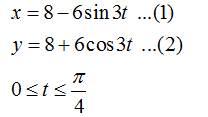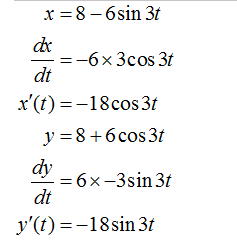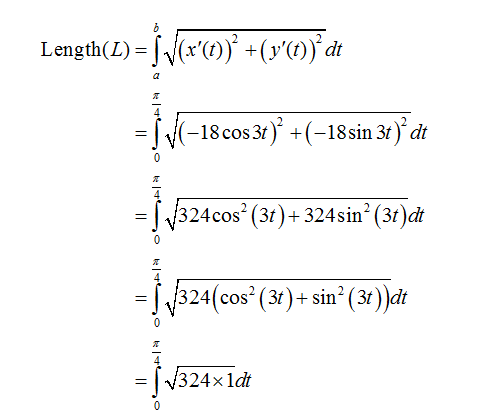# The parametric equations x= 8 − 6 sin 3t and y = 8 + 6 cos 3 t for 0 ≤ t ≤ π/4 represent a curve C.Find the length L of the curve C.L =

Question
1 views

The parametric equations x= 8 − 6 sin 3t and y = 8 + 6 cos 3 t for 0 ≤ t ≤ π/4 represent a curve C.

Find the length L of the curve C.
L =

check_circle

Step 1

Given:

Parametric equationsFormula usedStep 2

The length of the curve is given by

Differentiate equation (1) and (2)  with respect to tStep 3

Put all these values in the formula...

### Want to see the full answer?

See Solution

#### Want to see this answer and more?

Solutions are written by subject experts who are available 24/7. Questions are typically answered within 1 hour.*

See Solution
*Response times may vary by subject and question.
Tagged in